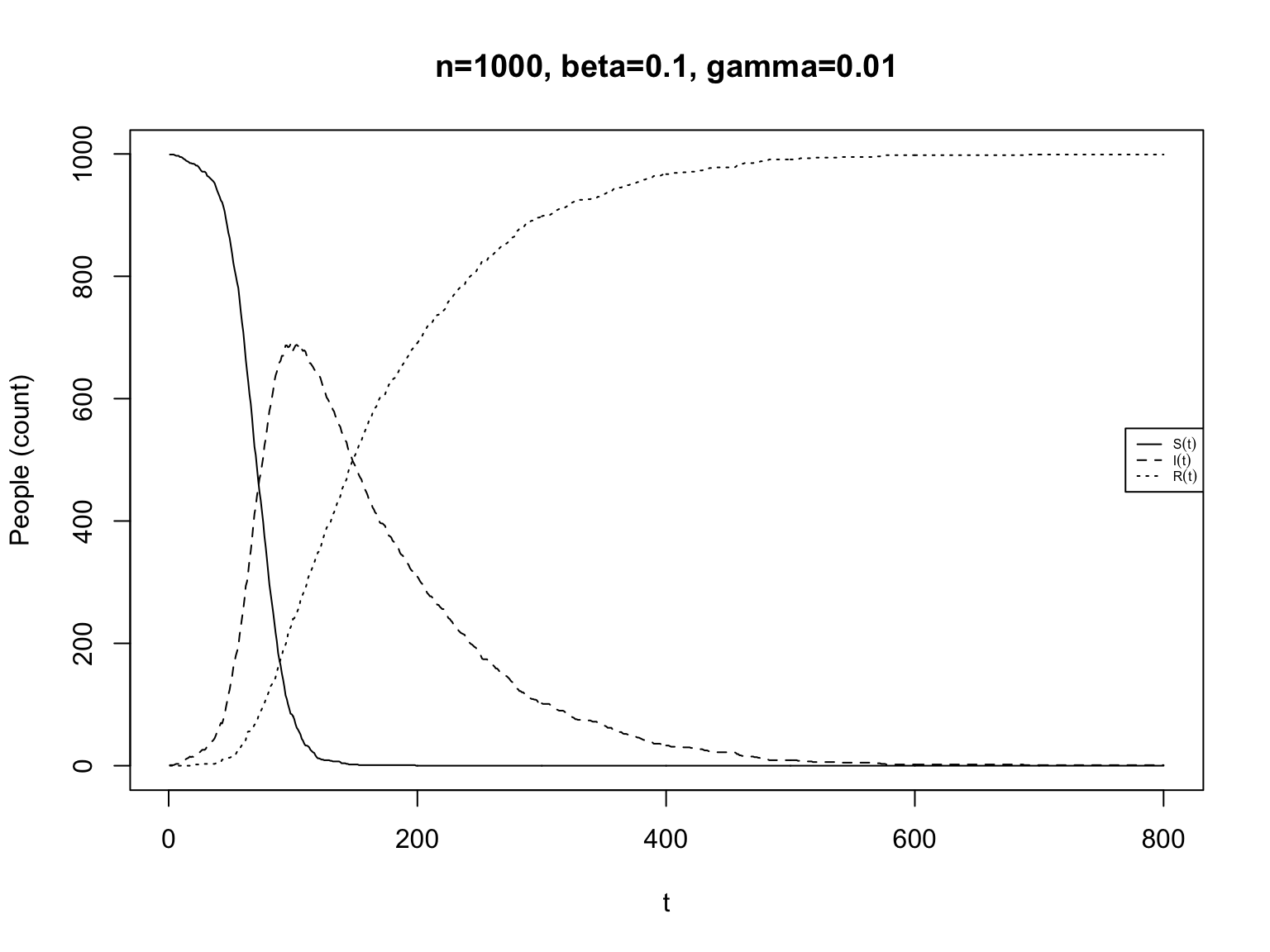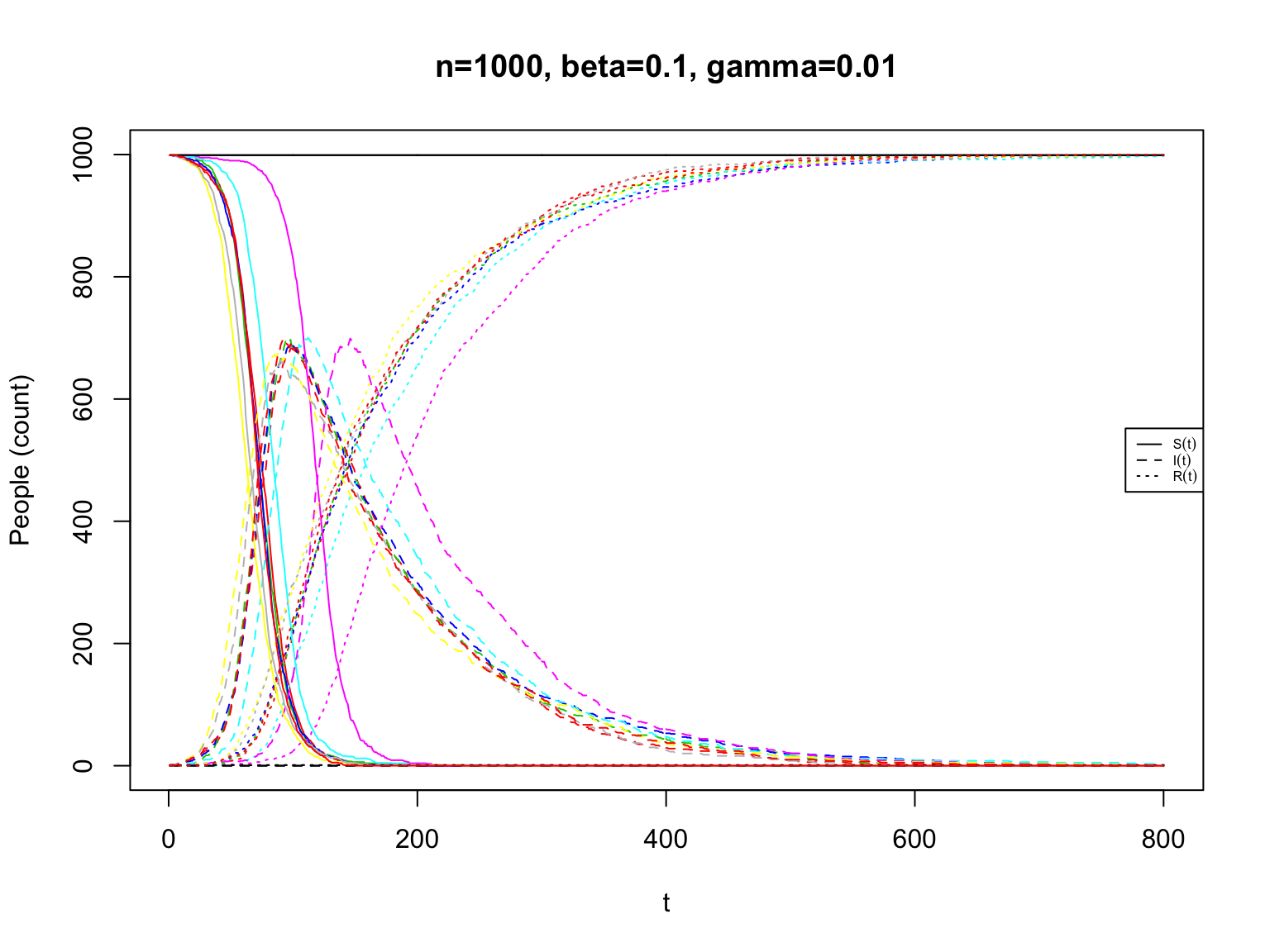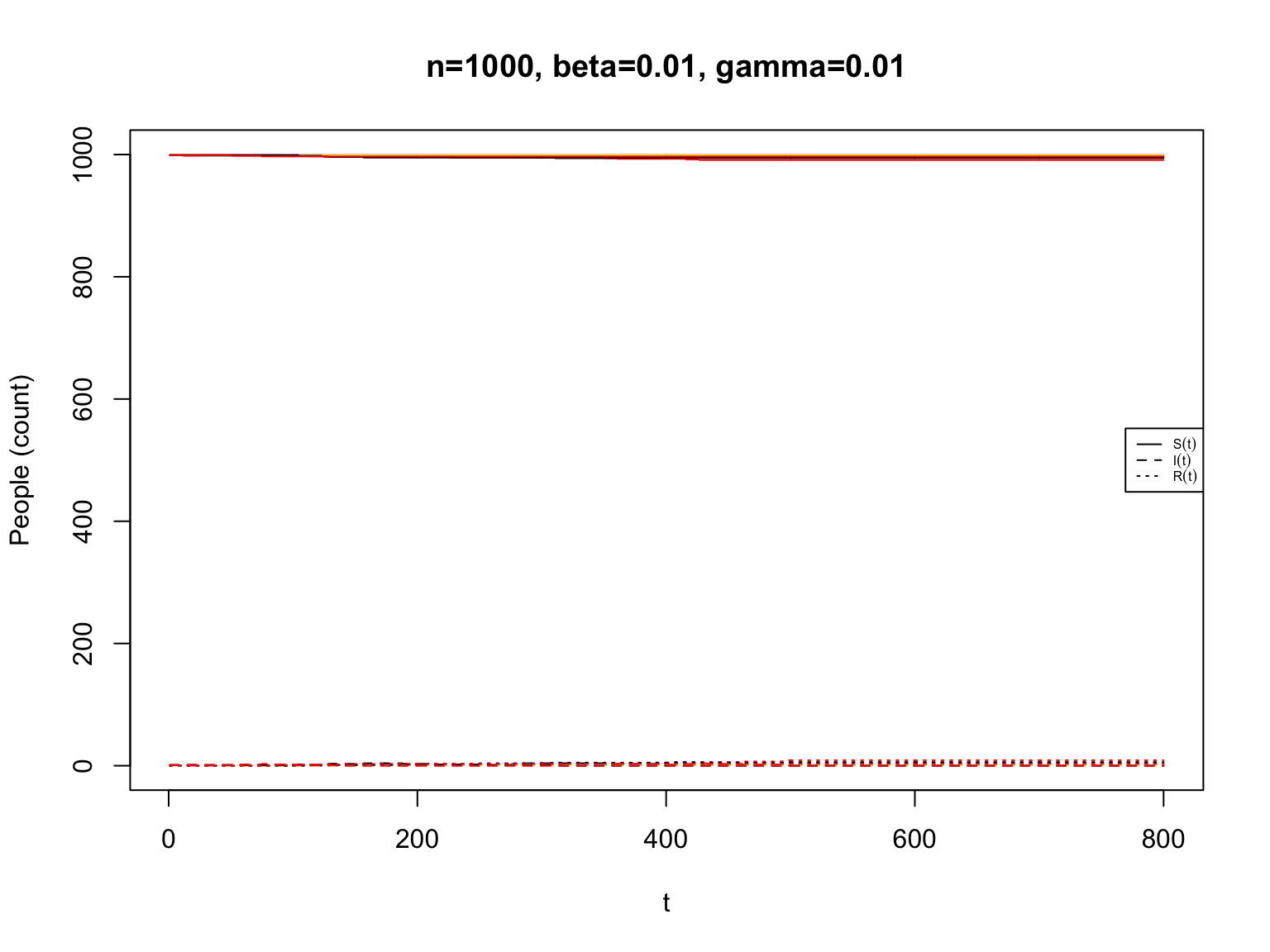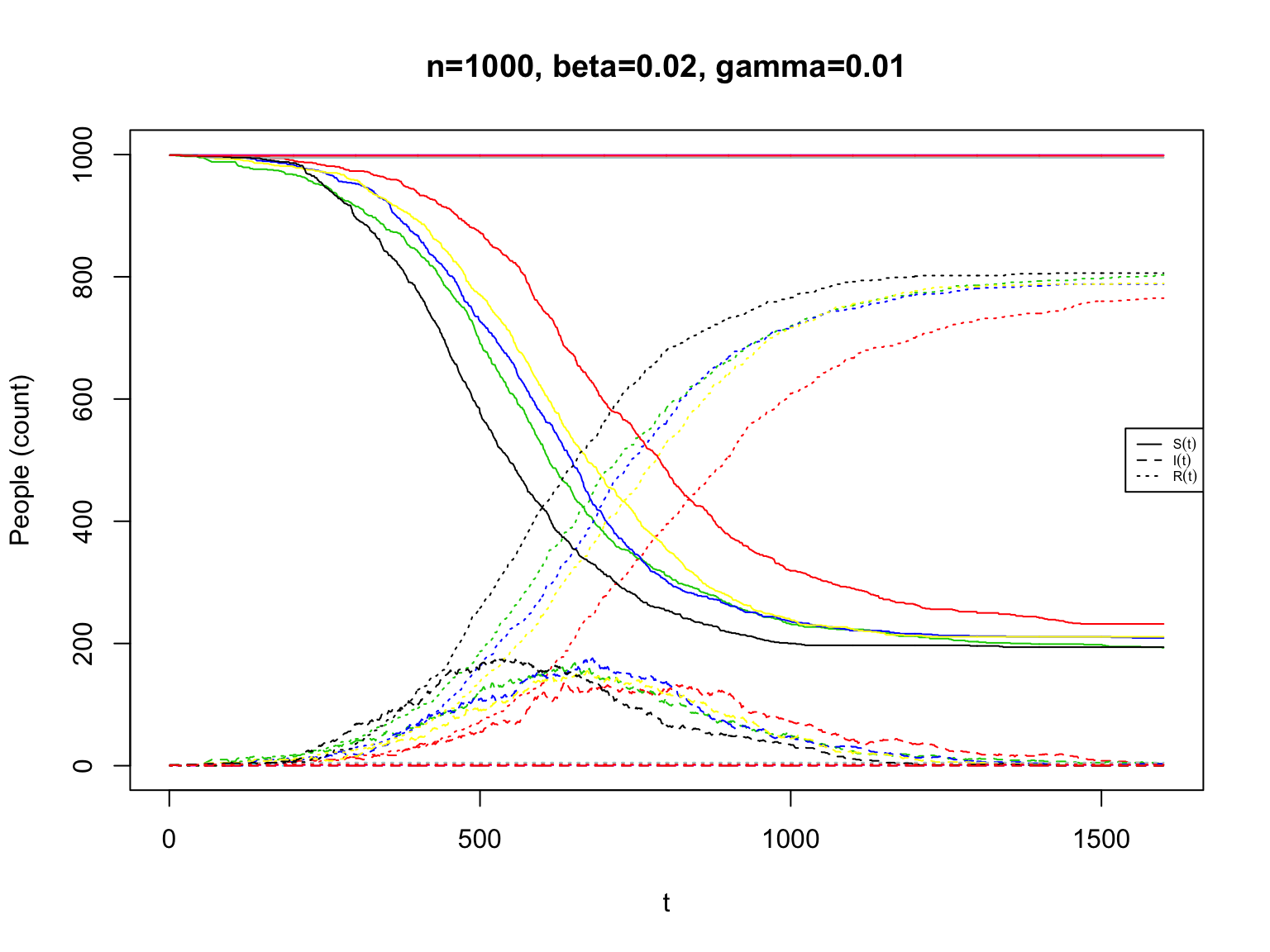# Epidemic Models

10 November 2020 (Lecture 20)

# Previously

• Markov models: the current state of the process fixes the distribution of the future; the past is irrelevant $\mathrm{Pr}\left( X(t+1), X(t+2), \ldots | X(t), X(t-1), \ldots X(0) \right) = \mathrm{Pr}\left( X(t+1), X(t+2), \ldots| X(t) \right)$
• Everything boils down to the transition probabilities, $$\mathrm{Pr}\left( X(t+1) = y|X(t) = x \right)$$
• Inference for Markov processes:
• Based on the likelihood, which we can write in terms of the transition probabilities
• Or based on aggregated counts

# “SIR” Epidemic Model

• Models the spread of a disease through a population
• Compartments: Every person can be in one of three states:
• Susceptible ($$S$$): not sick, could get sick
• Infectious ($$I$$): sick, can make others sick
• Removed ($$R$$): either recovered or dead; not sick, can’t get sick, can’t make others sick
• Contagion: When an $$S$$ meets an $$I$$, some probability of the $$S$$ also becoming $$I$$
• Removal: $$I$$s spontaneously change into $$R$$s
• Maybe after some random time
• Maybe at some steady rate per unit time
• Mixing or mass action: probability of an $$S$$ meeting an $$I$$ just depends on total numbers of $$S$$s, $$I$$s and $$R$$s

# Stochastic form of the SIR model (1)

• $$S(t) =$$ number of $$S$$s at time t, similarly for $$I(t)$$ and $$R(t)$$
• Total (initial) population is $$n$$
• Small time increment $$h$$, during which each $$S$$ person encounters $$k h$$ people
• The probability of contagion from an encounter between an $$S$$ and an $$I$$ is $$c$$
• Probability that a random person is infected is $$I(t)/n$$
• So the probability of an $$S$$ becoming $$I$$ is $$kch I(t)/n$$
• Strictly it’s $$1-(1-c I(t)/n)^{hk} \approx kch I(t)/n$$ for small $$h$$
• Number of new infections in time $$h$$ is $$\mathrm{Binom}(S(t), kch I(t)/n)$$
• If $$h$$ is very small but $$S(t)$$ is very large, this is approximately $$\mathrm{Poisson}(\frac{kch}{n} S(t) I(t))$$
• Every $$I$$ gets removed with probability $$\gamma h$$ so the number of removals in time $$h$$ is $$\mathrm{Binom}(I(t), \gamma h)$$
• If $$h$$ is very small but $$I(t)$$ is very large, this is approximately $$\mathrm{Poisson}(\gamma h I(t))$$

# Stochastic form of the SIR model (2)

• Over-all equations: $\begin{eqnarray} C(t+h) & = & \mathrm{Binom}(S(t), \frac{\beta}{n} h I(t))\\ D(t+h) & = & \mathrm{Binom}(I(t), \gamma h)\\ S(t+h) & = & S(t) - C(t+h)\\ I(t+h) & = & I(t) + C(t+h) - D(t+h)\\ R(t+h) & = & R(t) + D(t+h) \end{eqnarray}$

• This bundles up multiple parameters we don’t get to measure separately into some “effective” parameters $$\beta$$ and $$\gamma$$
• I left in the factors of $$h$$ and $$n$$ for reasons which will become clear later

# The SIR model is a Markov model

• The state variable $$X(t) = (S(t), I(t), R(t))$$
• Because $$S(t) + I(t) + R(t) = n$$, could eliminate one variable from the state (usually pick $$R(t)$$)
• The equations on the previous slide implicitly give the transition probabilities
• Explicit expressions are possible but tedious
• Markov property holds by assumption

# SIR is easy to simulate

sim.SIR <- function(n, beta, gamma, s.initial = n - 1, i.initial = 1, r.initial = 0,
T) {
stopifnot(s.initial + i.initial + r.initial == n)
states <- matrix(NA, nrow = 3, ncol = T)
rownames(states) <- c("S", "I", "R")
states[, 1] <- c(s.initial, i.initial, r.initial)
for (t in 2:T) {
contagions <- rbinom(n = 1, size = states["S", t - 1], prob = beta * states["I",
t - 1]/n)
removals <- rbinom(n = 1, size = states["I", t - 1], prob = gamma)
states["S", t] <- states["S", t - 1] - contagions
states["I", t] <- states["I", t - 1] + contagions - removals
states["R", t] <- states["R", t - 1] + removals
}
return(states)
}
• The code doesn’t need $$h$$ here because it’s (implicitly) taking $$h=1$$

• We’ll start by simulating the model to see what our assumptions imply (as one should always do)

# What One Simulation Looks Like# Multiple Simulations with the Same Settings• Most of these show similar behavior, but not quite the same
• Why do some runs have later outbreaks than others?
• What’s up with the horizontal black line at the top?

# What If We Make Contagion Harder?• The one initial $$I$$ gets removed before it can start an epidemic

# Maybe Not Quite That Much Harder?• Note the change on the horizontal axis
• Sometimes the epidemic never gets started, sometimes it takes off but doesn’t get everyone before dying out…
• Notice that when it does break out, it stops when about 80% of the population has been infected, not nearly-100% like in the first set of simulations